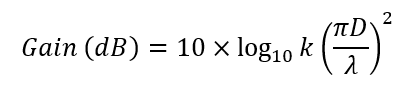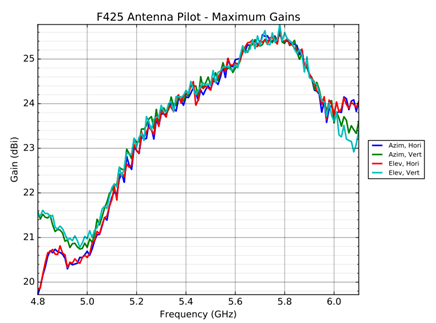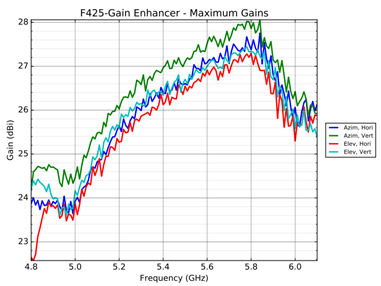Range Extender for Force 425

I would ask someone to explain to me.
If the additional surface that is double in this case gives a result of an additional 3 dBi, does that mean that the source parabola and the double-reflection waveguide are not optimized?
Is the original too wide? Is the gain of the F / B ratio antenna as declared?
As far as I know, with a properly optimized antenna, adding a parabola surface does not give amplification! What did I sleep over?

Regards

Hi,

Here is the math how antenna gain relate to dish diameter.For Force 425 dish, D= 450mm
w/ range extender D=650mm
Both case K is 40%.
One can calculate Gain for a given frequency to know how it varies w.r.t dish dia.

Here is the antenna gain measurements from lab,

Force 425 w/o RE:Force 425 w/ RE:2 Likes
1 Like

Math is ok.
The double surface of the parabola gives a gain of 3 dB
It’s all clear.
But it is not clear to me with a simple procedure to add a double surface and gain more by 3 dB. Without any intervention on the illuminator by moving the f / d or the angle of radiation of the illuminator. She can’t do that!
If it works as the charts say then it is not well optimized?
The original antenna without RE has too wide a radiation angle. The forward-backward relationship is disastrous.
Do you have those charts?
Regrds